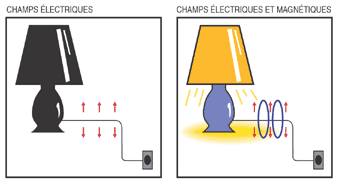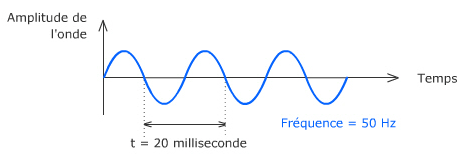# Electricity & Fields

Key points

Electric and magnetic fields are distinct concepts that were developed to explain the effects of electricity at a distance. What are the concepts to keep in mind when considering the potential relationship between 50 Hz electric and magnetic fields? See below…

### Electric and magnetic fields

Electric field (EF) is linked to voltage. The higher the voltage, the higher the EF. It is measured in Volt per meter (V/m).

Magnetic field (MF) is linked to current: The stronger the current, the higher the resulting MF. It is measured in amperes per meter (A/m) but tesla (T), the unit of magnetic flux, is generally preferred. A direct relationship exists between both units: it depends on the environment in which the field is moving. Practically, we often speak of the magnetic field, in T. Magnetic fields that we usually measure are in the order of microtesla (µT), or one millionth of Tesla.Figure 1a – Figure 1b

In practice: in Figure 1a, the lamp is off but connected to a voltage source, here the 230V electricity grid; so we can only measure EF. When the lamp is on (Fig. 1b), EF and MF exists. The intensity of the latter depends on the intensity of the current flowing, and hence the power of the lamp in this example.

### Frequencies

BBEMG research focuses on 50 Hz frequency, which is the frequency of the electricity network in Europe, generated at power plants and kept in the transmission and distribution of electricity to our homes. Our electrical devices operate at 50 Hz.

The electromagnetic spectrum covers a very wide range of frequencies (in Hz) and wavelengths (in m). 50 Hz fields are only a small part of the electromagnetic spectrum: visible light is also a part of the spectrum (frequency 385 THz to 750 THz, 1 THz = 1012 Hz), as well as radio waves from mobile phones (frequencies on the order of GHz, 1 GHz = 109 Hz) ) or X-rays (frequency around 1000 THz).

The alternating current that powers our electrical appliances has a frequency of 50 Hz, i.e. it performs 50 cycles per second: it alternates between a positive maximum value, zero, and a negative maximum value, then zero and so on (sinusoidal).### Voltage

The high voltage network carries electricity from the power plants. The low-voltage distribution system carries electricity to our homes. There are several high voltage levels (75, 150, 220 or 380 kV – thousands of volts) in Belgium and several low voltage levels; the voltage is lowered by transformers.

### Power & Current

Power is the amount of energy supplied or consumed per second. It is measured in watts (W). Our appliances are more or less energy efficient depending on the power they consume: for example, a 2000 W oven will consume more energy over a period of time that an economic bulb of 15 W.

The problem with electricity is that it cannot be stored. This means that the energy is to be supplied on consumer demand and therefore the electricity flowing through the network is not always at its maximum. The production of electricity in power plants must adapt to demand [*].

Power is linked to the intensity of the current, measured in ampere (A). One can say that for a certain voltage (for example a 150 kV line) the power and current vary in a similar manner in a day.

Electric current consists in a displacement of electrons in the material, i.e. in the copper or aluminum of which the electric cables are composed. In their movements, they bump to each other which causes an over-heating and a loss of their energy. This energy that is lost as heat, will not come to our homes and also imposes constraints on power infrastructure. To avoid these heat losses, it is necessary to increase the voltage: for the same power transported, if the voltage is increased (eg replacement of the 150 kV line into a 380 kV line), the intensity of the current will lower and electrons will make less movements.

### Units

Units
V Voltage in volt (V) t Time in second (s)
I Current intensity in ampere (A) L Length in meter (m)
q Electrical charge in coulomb (C) s Section in square meter (m²)
E Energy in joule (J) R Resistance in ohm (Ω)
P Power in watt (W) Z Impedance in ohm (Ω)
F Force in newton (N) Ω Resistivity in ohm . meter (Ω.m)
E Electric field in volt/meter (V/m) F Frequency in hertz (Hz)
H Magnetic field in ampere/meter (A/m) μ Magnetic permeability in henry/meter (H/m)
B Magnetic induction in tesla (T) λ Wavelength in meter (m)
Prefix of the power of 10
K Kilo one thousand: 103 m milli one thousandth: 10-3
M Mega one million: 106 µ micro one millionth: 10-6
G Giga one billion: 109 n nano one billionth: 10-9
T Tera thousand billion: 1012 p pico thousand billionth: 10-12